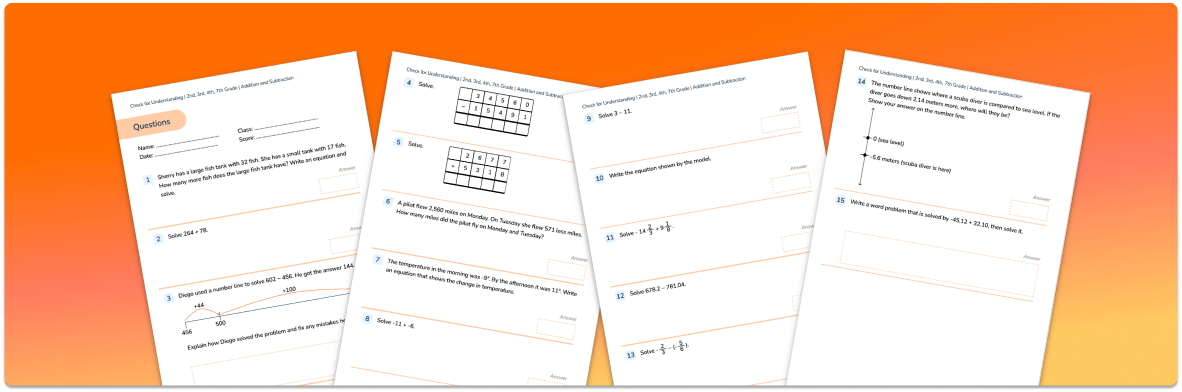Math teaching support you can trustone-on-one tutoring sessionsschools supported

[FREE] Themed Math Problems: Fall Term (Sep – Dec)

5th grade math problems and answers that link to key dates in the Fall Term, including Christmas!

# What Is A Number Sentence? Explained For Elementary School Teachers, Parents And Kids

In this post we will be answering the question “what is a number sentence?” and running through everything you need to know about this key concept for elementary school math! We’ve also got a selection of number sentence questions you can use to test out children’s skills, so make sure you scroll to the bottom of the post!

### What is a number sentence?

A number sentence is a combination of numbers and mathematical operations that children are often required to solve.

Examples of number sentences include:

32 + 57 = ?

5 x 6 = 10 x ?

103 + ? = 350

They will usually be composed of addition, subtraction, multiplication or division – or a combination of all four! Remember – You may consider the above simply as “sums”, but referring to them as this can be confusing for children because the word “sum” should only be used when discussing addition.Addition & Subtraction Check for Understanding

Support your teaching of the four operations by checking your students' understanding of addition and subtraction across 15 expertly-crafted questions!DOWNLOAD FREE

### When do children learn about number sentences?

From kindergarten, students will begin to read, write and interpret mathematical statements involving addition (+), subtraction (–) and equals (=) signs.

Number sentences build on what children will have already learned about number bonds.

As students progress, they will calculate mathematical statements for multiplication and division within the multiplication tables and write them using the multiplication (×), division (÷) and equals (=) signs.

Once they have mastered this, students will write and calculate mathematical statements for multiplication and division using the multiplication tables that they know, including two-digit numbers times one-digit numbers, using mental and progressing to formal written methods.

Students will continue to practice their mental recall of multiplication tables when they are calculating mathematical statements in order to improve fluency.

Students will write statements about the equality of expressions (for example, use the distributive law 39 × 7 = 30 × 7 + 9 × 7 and associative law (2 × 3) × 4 = 2 × (3 × 4).

Students will be expected to understand the terms factor, multiple and prime, square and cube numbers and use them to construct equivalence statements (for example, 4 x 35 = 2 x 2 x 35; 3 x 270 = 3 x 3 x 9 x 10 = 81 x 10).

They should also recognize mixed numbers and improper fractions and convert from one form to the other and write mathematical statements > 1 as a mixed number [for example, 2/5 + 4/5 = 6/5 = 1 and 1/5].

Wondering about how to explain other key math vocabulary to your children? Check out our Math Dictionary For Kids, or try these other math terms:

### Number sentence practice questions

1) Complete the following number sentences:

340 ÷ 7 = ____  remainder ____

____÷ 3 = 295 remainder 2

2) Here is a number sentence.

____ + 27 > 85

Circle all the numbers below that make the number sentence correct.

30       40       50       60       70

3) Write in the missing number.

Do you have students who need extra support in math?
Give your students more opportunities to consolidate learning and practice skills through personalized math tutoring with their own dedicated online math tutor.

Each student receives differentiated instruction designed to close their individual learning gaps, and scaffolded learning ensures every student learns at the right pace. Lessons are aligned with your state’s standards and assessments, plus you’ll receive regular reports every step of the way.

Personalized one-on-one math tutoring programs are available for:

The content in this article was originally written by primary school teacher Sophie Bartlett and has since been revised and adapted for US schools by elementary math teacher Christi Kulesza.##### Sophie Bartlett
Year 6 Teacher
Sophie teaches mixed age classes at a small school in central England. She is a self confessed grammar pedant and number nerd so we've welcomed her as a regular author and editor for Third Space Learning.
x#### Addition & Subtraction Check for Understanding

Support your teaching of the four operations by checking your students' understanding of addition and subtraction across 15 expertly-crafted questions!

#### Addition & Subtraction Check for UnderstandingSupport your teaching of the four operations by checking your students' understanding of addition and subtraction across 15 expertly-crafted questions!